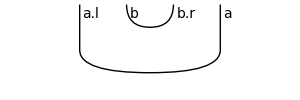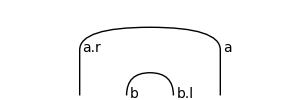# Diagram#

class discopy.grammar.pregroup.Diagram(inside, dom, cod, _scan=True)[source]#

Bases: `Diagram`

A pregroup diagram is a rigid diagram with `Word` boxes.

Parameters:
• inside (tuple[frobenius.Layer, ...]) – The layers of the diagram.

• dom (rigid.Ty) – The domain of the diagram, i.e. its input.

• cod (rigid.Ty) – The codomain of the diagram, i.e. its output.

Note

In order to define more general DisCoCat diagrams, pregroup diagrams subclass frobenius rather than rigid. Have fun with swaps and spiders!

Example

```>>> s, n = Ty('s'), Ty('n')
>>> Alice, Bob = Word('Alice', n), Word('Bob', n)
>>> loves = Word('loves', n.r @ s @ n.l)
>>> grammar = Cup(n, n.r) @ Id(s) @ Cup(n.l, n)
>>> sentence = grammar << Alice @ loves @ Bob
>>> print(sentence[:4])
Alice >> n @ loves >> n @ n.r @ s @ n.l @ Bob >> Cup(n, n.r) @ s @ n.l @ n
>>> from discopy import tensor
>>> ob = {s: 1, n: 2}
>>> ar = {Alice: [1, 0], loves: [0, 1, 1, 0], Bob: [0, 1]}
>>> F = tensor.Functor(ob, ar, dom=Category(), dtype=bool)
>>> assert F(sentence)
```
ty_factory#

alias of `Ty`

normal_form(**params)[source]#

Applies normal form to a pregroup diagram of the form `word @ ... @ word >> wires` by normalising words and wires seperately before combining them, so it can be drawn with `draw()`.

classmethod cups(left, right)[source]#

Construct a diagram of nested cups for types `left` and `right`.

Parameters:
• left (Ty) – The type left of the cup.

• right (Ty) – Its right adjoint.

Return type:

Diagram

Example

```>>> a, b = Ty('a'), Ty('b')
>>> Diagram.cups(a.l @ b, b.r @ a).draw(figsize=(3, 1),\
... margins=(0.3, 0.05), path='docs/_static/rigid/cups.png')
```classmethod caps(left, right)[source]#

Construct a diagram of nested caps for types `left` and `right`.

Parameters:
• left (Ty) – The type left of the cap.

• right (Ty) – Its left adjoint.

Return type:

Diagram

Example

```>>> a, b = Ty('a'), Ty('b')
>>> Diagram.caps(a.r @ b, b.l @ a).draw(figsize=(3, 1),\
... margins=(0.3, 0.05), path='docs/_static/rigid/caps.png')
```braid_factory#

alias of `Swap`

cap_factory#

alias of `Cap`

cup_factory#

alias of `Cup`

factory#

alias of `Diagram`

spider_factory#

alias of `Spider`